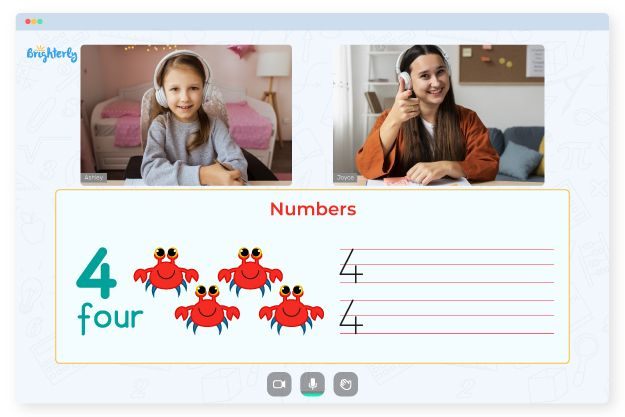# Number 14 Worksheets

Study the number 14 in a fun way using number 14 worksheets that are ideal for kindergarteners or grade 1 students who learn to count and recognize a set of numbers.

You can use number 14 worksheets for preschoolers to boost your child’s addition skills. These number worksheets help kids practice adding double-digit numbers.### Number 14 Worksheets PDF

Number 14 Worksheet### Number 14 Worksheets PDF

Number 14 Worksheet Preschool### Number 14 Worksheets PDF

Number 14 Worksheets For Preschoolers### Number 14 Worksheets PDF

Number 14 Tracing Worksheet

Worksheets are designed to help kids add the provided numbers with the number 14. For example, 14 +3 =17.

Kids can practice solving additional problems using the number 14 to improve knowledge of arithmetic concepts.

## Number 14 Subtraction Worksheets

When you engage your children in practicing subtraction problems using worksheets, they will improve their performance and score good marks in the subject.

In a number 14 subtraction worksheet, the young ones need to subtract the provided numbers with the number 14. For example, 18-14=4.

Math for Kids

Is Your Child Struggling With Math?
1:1 Online Math Tutoring## Number 14 Bond Worksheets

The use of number bonds is a great way for youngsters to learn how to use basic pairs of numbers that equal a specified number. Worksheets like this one combine numbers whose sum is 14, such as 12 + 2, 14 + 0, 7 + 7, 10 + 4.

Using a number 14 bond worksheet, you can help your child learn the structures inherent in the basic addition facts. The number bond helps learners approach addition facts from a conceptual point of view rather than memorizing numbers randomly or in sets of +1 facts, +2 facts, and more.

The numbers in the following sequence can be used to introduce your youngster to the 14-number bond:

14+0=14

13+1=14

12+2=14

11+3=14

10+4=14

You can teach your child how to draw the additional bond pattern and then have a kid use different colored pencils to draw it on a piece of blank paper. A child will be able to study while having fun and figuring out the pattern at the same time.

Help your child write the numbers from 14 to 0 vertically at the beginning. Preparing a youngster to write the other numbers in order until they reach 20 or 100 will be easier by using the number 14 bond.

### Numbers Worksheets

Struggling with Numbers?• Does your child struggle to grasp numbers concepts?
• Try studying with an online tutor.

Is your child having difficulties with numbers concepts? Start lessons with an online tutor.